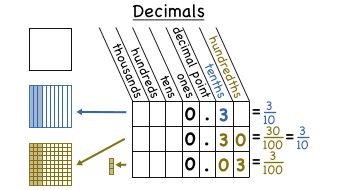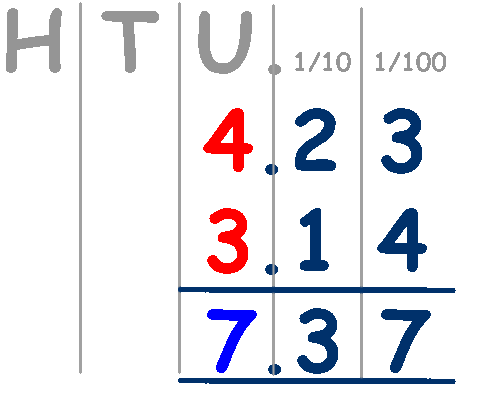# Decimals

## Lesson Intention

We are going to learn about decimalsSuccess Criteria – you will know you have been successful when you can:

# Accurately 80%+ add and subtract using decimals.

# Order decimals with 80%+ accuracy.

# Multiply decimals by powers of 10.

# Divide with decimals (accuracy of 60%)Ordering Decimals

Decimal Ordering Game

Ordering Decimals Quiz

Maths Dictionary – Multiplying Decimals

Quiz – Multiplying Decimals

Dictionary – Dividing Decimals

Dividing Decimals Practise

Quiz – Dividing Decimals

## 8 thoughts on “Decimals”

1.Dukeman says:

I can Accurately 80%+ add and subtract using decimals, order decimals with 80%+ accuracy, i need help with multiplying decimals by powers of 10.

2.Joshua says:

Decimals what I can do- I can add and subtract decimals with 80% accuracy, ordering decimals with 80% accuracy but because I don’t know what powers of 10 is so i’ll say that I might need help with multiplying decimals by the power of 10.

3.Tegan says:

I can:
Order decimals with 80 % accuracy and multiply decimals by the power of ten.

I need to work on:
Add and subtract decimals with 80% accuracy.

4.jammy says:

# Accurately (80%+) add & subract using decimals.
Yes, with about 95% accuracy 🙂

# Order decimals with at least 80%+ accuracy.
Yep, easily with 100% accuracy.

# I still need to work on multiplying decimals by the power of 10 🙂

5.Lachlan says:

I am getting 80% accuracy but i need to work on my simlifying because if i do not simplify i will get the question right and will not get 100% or 90%

6.Tegan says:

I can order decimals with 80% accuracy and multiply decimals by the power of ten.

I need to work on adding and subtracting the decimals with 80% accuracy.

7.Abbey says:

What I know:

Decimals: I can accurately order decimals with 80%+ accuracy, as well as adding and subtracting decimals. I don’t actually know what multiply decimals by powers of 10 means, I may know how to do it I just don’t think I called it that. Otherwise I think I am doing OK but I may just need a little more help with decimals, as that wouldn’t hurt a bit.

From Abbey

8.Freyja says:

Decimals -I know how to order decimals with 80%+ accuracy. I alao know how to multiply decimals by powers of 10. I need some help with subtracting using decimals, but I can add decimals.